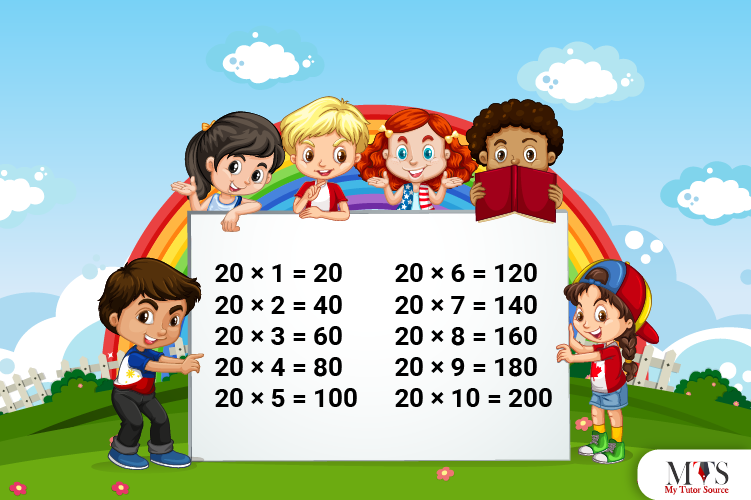# Multiplication Table of 20 – Tips to Memorize 20 Times Table & Example QuestionsPick any application of mathematics; multiplication tables would be its essential tool. Learning and memorizing tables helps perform time-consuming tasks in seconds, break down concepts, heighten confidence, and encourage mental arithmetic skills in students. Whether you have to perform a competitive exam question or do daily tasks, multiplication table knowledge is necessary. Students struggling with mathematics or multiplication tables should always go for extra help. Taking sessions with a private math tutor not only helps students attain good grades but also boosts their confidence.

Memorizing 20 times table is necessary for elementary school students. In this article, we have provided a table of 20 with tips for memorization and a few solved examples for practice.

Let us learn the table of 20!

Table of 20

Table of 20 is one of the easy tables for students. 20 is an even number; that’s why the product of its multipliers is also in even numbers. Look at the table of 20 given below.

20 x 1 = 20
20 x 2 = 40
20 x 3 = 60
20 x 4 = 80
20 x 5 = 100
20 x 6 = 120
20 x 7 = 140
20 x 8 = 160
20 x 9 = 180
20 x 10 = 200
20 x 11 = 220
20 x 12 = 240
20 x 13 = 260
20 x 14 = 280
20 x 15 = 300
20 x 16 = 320
20 x 17 = 340
20 x 18 = 360
20 x 19 = 380
20 x 20 = 400

## Method to Read Table of 20

In this section, you will find the table of 20 written in words. This section is to help you read and memorize the table.

One time twenty is twenty (20)
Two times twenty are forty (40)
Three times twenty are sixty (60)
Four times twenty are eighty (80)
Five times twenty are one hundred (100)
Six times twenty are one hundred and twenty (120)
Seven times twenty are one hundred and forty (140)
Eight times twenty are one hundred and sixty (160)
Nine times twenty are one hundred and eighty (180)
Ten times twenty are two hundred (200)
Eleven times twenty are two hundred and twenty (220)
Twelve times twenty are two hundred and forty (240)
Thirteen times twenty are two hundred and sixty (260)
Fourteen times twenty are two hundred and eighty (280)
Fifteen times twenty are three hundred (300)
Sixteen times twenty are three hundred and twenty (320)
Seventeen times twenty are three hundred and forty (340)
Eighteen times twenty are three hundred and sixty (360)
Nineteen times twenty are three hundred and eighty (380)
Twenty times twenty are four hundred (400)

## Tips for Memorizing Multiplication Table of 20

There are many tips to memorize the 20 times table, but we have mentioned the easiest one here. Recall the multiplication table of 2 and add 0 at its multiples’ unit’s place. By doing so, you will obtain the multiples of 20 times table. Go through the table below to see how this tip works:

Another tip for memorizing the 20 times table is to use the repeated addition method. Add 20 into the product of multipliers to generate the next product.

For example,

20 x 1 = 20
20 x 2 = 20 + 20 = 40
20 x 3 = 20 + 20 + 20 = 60
20 x 4 = 20 + 20 + 20 + 20 = 80
20 x 5 = 20 + 20 + 20 + 20 + 20 = 100
20 x 6 = 20 + 20 + 20 + 20 + 20 + 20 = 120 and so on.

### Example Question of Table of 20

#### Question: Find the value of 20 times 9 minus 20 times 5?

Solution:

Given that,
20 times 9 minus 20 times 5
Mathematically it is written as
= 20 x 9 – 20 x 5
= 180 – 100
= 80
Thus, the value of 20 times 9 minus 20 times 5 is 80.

___ x 20 = 120

20 x ___ = 80

___ x ___ = 240

___ x 17 = ___

___ x 2 = 40

20 x ___ = 380

___ x 9 = ___

___ x ___ = 140

___ x 20 = 300

___ x ___ = 180

#### Question: Evaluate 20 times 7 and 20 times 17?

Solution:

20 times 7 and 20 times 17
= 20 x 7 and 20 x 17
= 140 and 340
Hence, the value of 20 times 7 and 20 times 17 is 140 and 340, respectively.

#### Question: Sandy wants to buy 15 chocolates for her 15 friends. If the cost of one chocolate is 20 \$, using the table of 20, estimate the cost of 15 chocolates?

Solution:

Given that,
Total chocolates = 15
Cost of one chocolate = 20\$
Using table of 20
Cost of 15 chocolates = 15 x 20 = 300
Hence, the cost of 15 chocolates is 300\$.

#### Question: Find a number before and after 20 times 6?

Solution:

Given that,
20 times 6
In mathematical form,
= 20 x 6
= 120
The number before 120 = 120 – 1 = 119
The number after 120 = 120 + 1 = 121
Hence, a number before and after 20 times 6 is 119 and 121, respectively.

### Find Top Tutors in Your AreaWith over 3 years of experience in teaching, Chloe is very deeply connected with the topics that talk about the educational and general aspects of a student's life. Her writing has been very helpful for students to gain a better understanding of their academics and personal well-being. I’m also open to any suggestions that you might have! Please reach out to me at chloedaniel402 [at] gmail.com